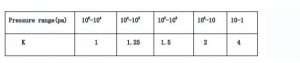working time of Vacuum pump calculation formula

Generally speaking, we are required to calculate the required time from initial pressure to required pressure.So we should calculate it according to initial pressure, required pressure, volume of vacuum chamber.conversely,we could also select suitable pump according to initial pressure, required pressure, volume of vacuum chamber and time (of course, the selection of the vacuum pump is an integrated process involving factors such as relevant experience).

Computational formula is as below:

T = kV / s × ln (P1 / P2)

T is pumping time (s)

V is the volume of vacuum chamber (L)

S is the pumping speed (L / S)

P1 is initial pressure (Pa)

P2 is required pressure (Pa)For example: Volum of vacuum chamber is 10m3, ultimate pressure is 10pa, working pressure is 200pa，main pump H-150G piston pump（150l/s），how long it will reach to required ultimate pressure?

T=kV/s×ln(P1/P2)  ln2=0.67  ln10=2.3

T1=1.5*10000/150 *ln(200/100)=67

T2=2*10000/150*ln(100/10)=306

T=T1+T2=67+306=373(s)

So it takes about 373second to reach to ultimate pressure.

Tags: Piston pump

Edited by Nancy Zhang / EVP vacuum solution

Related videos

— Vacuum pump for alcohol concentration in the food industry

Posted on Fri, 18 Oct 2019 06:59:48 +0000

— High pumping speed screw vacuum pump

Posted on Fri, 18 Oct 2019 05:45:29 +0000

— Where to buy the best vacuum pump

Posted on Fri, 18 Oct 2019 05:34:13 +0000

— vacuum pump for mould industry

Posted on Fri, 18 Oct 2019 05:28:33 +0000

— Best vacuum pump application

Posted on Fri, 18 Oct 2019 05:23:15 +0000

— Vacuum pump for vacuum preservation

Posted on Fri, 18 Oct 2019 05:16:58 +0000